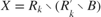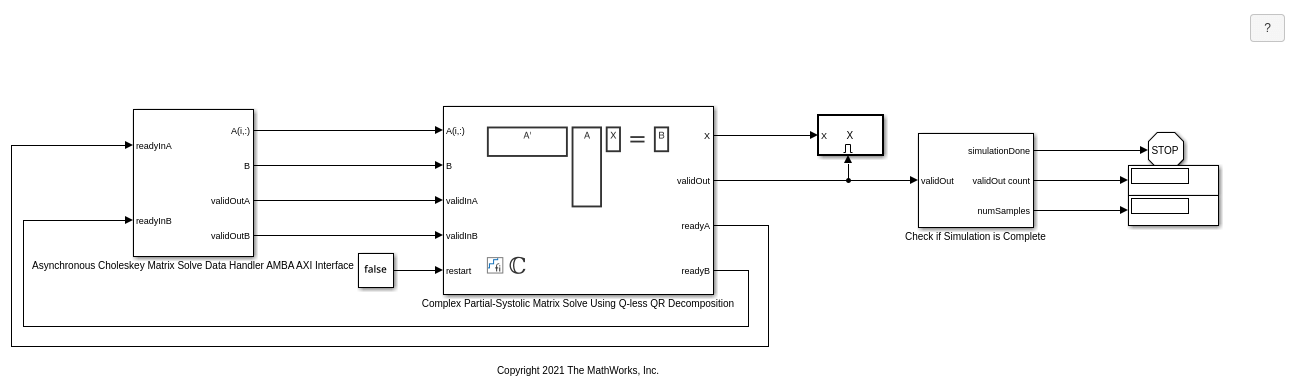# Implement Hardware-Efficient Complex Partial-Systolic Matrix Solve Using Q-less QR Decomposition

This example shows how to implement a hardware-efficient solution to the complex-valued matrix equation A'AX=B using the Complex Partial-Systolic Matrix Solve Using Q-less QR Decomposition block.

### Forward and Backward Substitution

When an upper triangular factor is ready, then forward and backward substitution are computed with the current input B to produce output X.

``

### Define Matrix Dimensions

Specify the number of rows in matrix A, the number of columns in matrix A and rows in B, and the number of columns in matrix B.

```m = 30; % Number of rows in A n = 10; % Number of columns in A and rows in B p = 1; % Number of columns in B numInputs = 3; % Number of A and B matricies ```

### Generate Matrices

For this example, use the helper function `complexRandomQlessQRMatrices` to generate random matrices A and B for the problem A'AX=B. The matrices are generated such that the real and imaginary parts of the elements of A and B are between -1 and +1, and A is full rank.

```rng('default') [A,B] = fixed.example.complexRandomQlessQRMatrices(m,n,p); if numInputs > 1 for i = 2:numInputs [Atemp,Btemp] = fixed.example.complexRandomQlessQRMatrices(m,n,p); A = cat(3,A,Atemp); B = cat(3,B,Btemp); end end ```

### Select Fixed-Point Data Types

Use the helper function `complexQlessQRMatrixSolveFixedpointTypes` to select fixed-point data types for input matrices A and B, and output X such that there is a low probability of overflow during the computation.

The real and imaginary parts of the elements of A and B are between -1 and 1, so the maximum possible absolute value of any element is sqrt(2).

```max_abs_A = sqrt(2); % Upper bound on max(abs(A(:)) max_abs_B = sqrt(2); % Upper bound on max(abs(B(:)) precisionBits = 24; % Number of bits of precision T = fixed.complexQlessQRMatrixSolveFixedpointTypes(m,n,max_abs_A,max_abs_B,precisionBits); A = cast(A,'like',T.A); B = cast(B,'like',T.B); OutputType = fixed.extractNumericType(T.X); ```

### Open the Model

```model = 'ComplexPartialSystolicQlessQRMatrixSolveModel'; open_system(model); ```### AMBA AXI Handshaking Process

The Data Handler subsystem in this model takes complex matrices A and B as inputs. It sends rows of A and full matrix of B to the QR Decomposition block using the AMBA AXI handshake protocol. The `validIn` signal indicates when data is available. The `ready` signal indicates that the block can accept the data. Transfer of data occurs only when both the `validIn` and `ready` signals are high. You can set delays for the feeding in rows of A and the feeding in of B matrices in the Data Handler to emulate the processing time of the upstream block. `validInA` and `validInB` remain high when `aDelay` and `bDelay` are set to `0` becuase this indicates the Data Handler always has data available. When all matrices A and B are sent, the Data Handler loops back to the first A and B matrices.

### Asynchronous Matrix Solver

This block operates asynchronously. First, Q-less QR decomposition is performed on the input A matrix and the resulting R matrix is put into a buffer. Then, the Forward Backward Substitute block uses the input B matrix and the buffered R matrix to compute R'RX = B. Because the R and B matrices are stored separately in buffers, the upstream Q-less QR decomposition block and the downstream Forward Backward Substitute block can run independently. The Forward Backward Substitute block starts processing when the first R and B matrices are available. Then it runs continuously using the latest buffered R and B matrices, regardless of the status of the Q-less QR Decomposition block. For example, if the upstream block stops providing A and B matrices, the Forward Backward Substitute block continues to generate the same output using the last pair of R and B matrices.

The Data Handler sends A and B matrices to the QR decomposition block iteratively. After sending out the last A matrix, the Data Handler resets its internal counter and sends out first A matrix. The B matrix is handled in a similar fashion.

### Set Variables in the Model Workspace

Use the helper function `setModelWorkspace` to add the variables defined above to the model workspace. These variables correspond to the block parameters for the Complex Partial-Systolic Matrix Solve Using Q-less QR Decomposition block.

```numOutputs = 1; % Number of recorded outputs aDelay = 1; % Delay of clock cycles between feeding in rows of A bDelay = 1; % Delay of clock cycles between feeding in B matrices fixed.example.setModelWorkspace(model,'A',A,'B',B,'m',m,'n',n,'p',p,... 'regularizationParameter',0,... 'aDelay',aDelay,'bDelay',bDelay,... 'numOutputs',numOutputs,'OutputType',OutputType); ```

### Simulate the Model

```out = sim(model); ```

### Construct the Solution from the Output Data

The Complex Partial-Systolic Matrix Solve Using Q-less QR Decomposition block outputs matrix X at each time step. When a valid result matrix is output, the block sets `validOut` to true.

```X = out.X; ```

### Verify the Accuracy of the Output

To evaluate the accuracy of the Complex Partial-Systolic Matrix Solve Using Q-less QR Decomposition block, compute the relative error. Choose the last output of the simulation.

```X = double(X(:,:,end)); ```

Synchronize the last output X with the input by finding the inputs A and B that produced it.

```A = double(A); B = double(B); relative_errors = zeros(size(A,3),size(B,3)); for k = 1:size(A,3) for g = 1:size(B,3) relative_errors(k,g) = norm(A(:,:,k)'*A(:,:,k)*X - B(:,:,g))/norm(B(:,:,g)); end end [AUsed,Bused] = find(relative_errors==min(relative_errors,[],'all')) %#ok<NOPTS> relative_error = norm(double(A(:,:,AUsed)'*A(:,:,AUsed)*X - B(:,:,Bused)))/norm(double(B(:,:,Bused))) %#ok<NOPTS> ```
```AUsed = 1 Bused = 2 relative_error = 6.1162e-05 ```